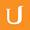## Linear Regression with Gradient Descent - Intro to Data Science

• 0:01 - 0:04
When performing linear regression, we have a number of data points.
• 0:04 - 0:09
Let's say that we have 1, 2, 3 and so on up through M data points.
• 0:09 - 0:12
Each data point has an output variable, Y,
• 0:12 - 0:15
and a number of input variables, X1 through X N.
• 0:16 - 0:20
So in our baseball example Y is the lifetime number of home runs.
• 0:20 - 0:24
And our X1 and XN are things like height and weight.
• 0:24 - 0:28
Our one through M samples might be different baseball players.
• 0:28 - 0:33
So maybe data point one is Derek Jeter, data point two is Barry Bonds, and
• 0:33 - 0:35
data point M is Babe Ruth.
• 0:35 - 0:38
Generally speaking, we are trying to predict the values of
• 0:38 - 0:42
the output variable for each data point, by multiplying the input variables by
• 0:42 - 0:46
some set of coefficients that we're going to call theta 1 through theta N.
• 0:46 - 0:49
Each theta, which we'll from here on out call the parameters or
• 0:49 - 0:53
the weights of the model, tell us how important an input variable is
• 0:53 - 0:56
when predicting a value for the output variable.
• 0:56 - 0:57
So if theta 1 is very small,
• 0:57 - 1:02
X1 must not be very important in general when predicting Y.
• 1:02 - 1:04
Whereas if theta N is very large,
• 1:04 - 1:07
then XN is generally a big contributor to the value of Y.
• 1:07 - 1:10
This model is built in such a way that we can multiply each X by
• 1:10 - 1:14
the corresponding theta, and sum them up to get Y.
• 1:14 - 1:17
So that our final equation will look something like the equation down here.
• 1:17 - 1:20
Theta 1 plus X1 plus theta 2 times X2,
• 1:20 - 1:24
all the way up to theta N plus XN equals Y.
• 1:24 - 1:27
And we'd want to be able to predict Y for each of our M data points.
• 1:28 - 1:32
In this illustration, the dark blue points represent our reserve data points,
• 1:32 - 1:35
whereas the green line shows the predictive value of Y for
• 1:35 - 1:38
every value of X given the model that we may have created.
• 1:38 - 1:41
The best equation is the one that's going to minimize the difference across all
• 1:41 - 1:44
data points between our predicted Y, and our observed Y.
• 1:45 - 1:49
What we need to do is find the thetas that produce the best predictions.
• 1:49 - 1:53
That is, making these differences as small as possible.
• 1:53 - 1:57
If we wanted to create a value that describes the total areas of our model,
• 1:57 - 1:58
we'd probably sum up the areas.
• 1:58 - 2:02
That is, sum over all of our data points from I equals 1, to M.
• 2:02 - 2:05
The predicted Y minus the actual Y.
• 2:05 - 2:08
However, since these errors can be both negative and
• 2:08 - 2:12
positive, if we simply sum them up, we could have
• 2:12 - 2:17
a total error term that's very close to 0, even if our model is very wrong.
• 2:17 - 2:21
In order to correct this, rather than simply adding up the error terms,
• 2:21 - 2:23
we're going to add the square of the error terms.
• 2:23 - 2:27
This guarantees that the magnitude of each individual error term,
• 2:27 - 2:30
Y predicted minus Y actual is positive.
• 2:31 - 2:34
Why don't we make sure the distinction between input of variables and
• 2:34 - 2:35
output of variables is clear.
Title:
Linear Regression with Gradient Descent - Intro to Data Science
Video Language:
English
Team:Udacity
Project:
ud359: Intro to Data Science
Duration:
02:36Udacity Robot edited English subtitles for Linear Regression with Gradient Descent - Intro to Data ScienceCogi-Admin edited English subtitles for Linear Regression with Gradient Descent - Intro to Data Science

# English subtitles

## Revisions Compare revisions

• API
Udacity Robot
• API